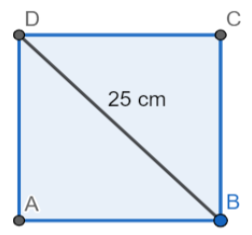Find the area of the square, the length of whose diagonal is 25 cm.

# Find the area of the square, the length of whose diagonal is 25 cm.

1. A
312.5 sq.cm
2. B
317.5 sq.cm
3. C
318.5 sq.cm
4. D
319.5 sq.cm

Fill Out the Form for Expert Academic Guidance!l

+91

Live ClassesBooksTest SeriesSelf Learning

Verify OTP Code (required)

### Solution:

Concept- We are aware that if a square's side length is equal to x, then the square's area is equal to. Now that we have the idea, we can calculate the required square's area.It is evident from the diagram that the square's diagonal measures 25 cm in length.
Area of square =Hence, the correct answer is option 1.

## Related content

 Area of Square Area of Isosceles Triangle Pythagoras Theorem Triangle Formula Perimeter of Triangle Formula Area Formulae Volume of Cone Formula Matrices and Determinants_mathematics Critical Points Solved Examples Type of relations_mathematics+91

Live ClassesBooksTest SeriesSelf Learning

Verify OTP Code (required)